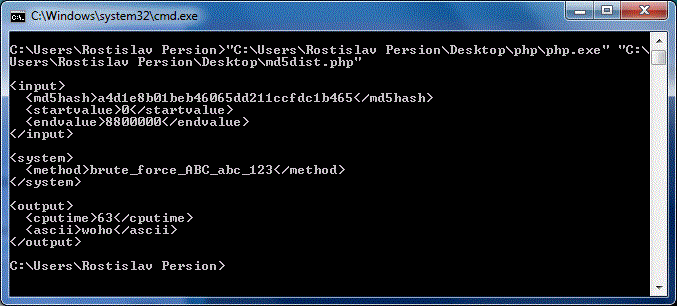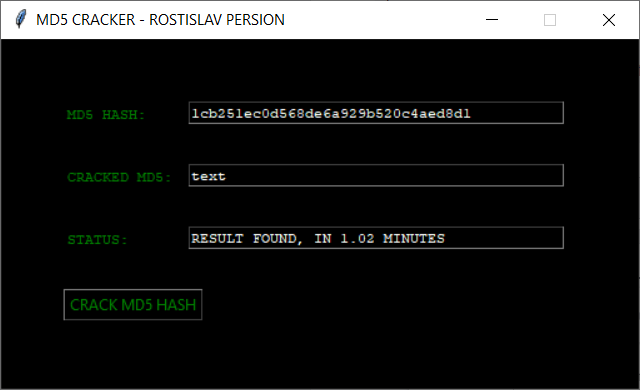# C:\> Rostislav Persion's Projects

.:: Distributed MD5 Cracker ::.
Distributed MD5 Cracker (PHP and now PYTHON)This program is a brute force MD5 hash cracker. It generates strings and encodes them. Then it compares the encoding to the MD5 hash. If the encoding is the same as the hash, the program displays the result.

SOFTWARE WRITTEN IN PHP
`   1:  <?php`
`   2:   `
`   3:  //get user input`
`   4:  \$mdhash = "76175b4492147d59fa0376a44bf9ead7";    //\$_GET['mdhash'];`
`   5:  \$numstart = 0;                                    //\$_GET['start'];`
`   6:  \$numend = 8800000;                                //\$_GET['end'];`
`   7:  \$iffoundemail = "seeplusplus@gmail.com";        //\$_GET['email'];`
`   8:   `
`   9:  //show user input`
`  10:  print("\n<input>\n");`
`  11:  print("  <md5hash>" . \$mdhash . "</md5hash>\n");`
`  12:  print("  <startvalue>" . \$numstart . "</startvalue>\n");`
`  13:  print("  <endvalue>" . \$numend . "</endvalue>\n");`
`  14:  print("</input>\n");`
`  15:   `
`  16:  //show system settings`
`  17:  print("\n<system>\n");`
`  18:  print("  <date>" . date("d/m/y", time()) . "</date>\n");`
`  19:  print("  <time>" . date("H:i:s", time()) . "</time>\n");`
`  20:  print("  <method>brute_force_ABC_abc_123</method>\n");`
`  21:  print("</system>\n\n");`
`  22:   `
`  23:  //define character set`
`  24:  \$lex = Array(`
`  25:  " ","a","b","c","d","e","f","g","h","i","j","k","l",`
`  26:  "m","n","o","p","q","r","s","t","u","v","w","x","y",`
`  27:  "z","A","B","C","D","E","F","G","H","I","J","K","L",`
`  28:  "M","N","O","P","Q","R","S","T","U","V","W","X","Y",`
`  29:  "Z","0","1","2","3","4","5","6","7","8","9");`
`  30:   `
`  31:  //count character set`
`  32:  \$numbase = count(\$lex);`
`  33:   `
`  34:  //declare working variables`
`  35:  \$res=0; \$powres=0; \$mant=0; \$num=0; \$num2=0;\$found=false;`
`  36:   `
`  37:  //main loop`
`  38:  \$t1 = time();`
`  39:  for (\$num2=\$numstart;\$num2<\$numend;\$num2++)`
`  40:  {`
`  41:      \$num = \$num2;`
`  42:      \$mant = floor(log(\$num,\$numbase));`
`  43:      \$strres = "";        `
`  44:      while (\$mant >= 0)`
`  45:      {`
`  46:          \$powres = pow(\$numbase, \$mant);`
`  47:          \$res = floor(\$num / \$powres);`
`  48:          \$strres .= \$lex[\$res];`
`  49:          \$num -= floor(\$res * \$powres);`
`  50:          \$mant--;`
`  51:      }`
`  52:      if (md5(\$strres) == \$mdhash)`
`  53:      {`
`  54:          \$found = true;`
`  55:          break;`
`  56:      }`
`  57:  }`
`  58:  \$t2 = time();`
`  59:   `
`  60:  //show result`
`  61:  if (\$found)`
`  62:  {`
`  63:      print("<output>\n");`
`  64:      print("  <cputime>" . (\$t2 - \$t1) . "</cputime>\n");`
`  65:      print("  <ascii>" . \$strres . "</ascii>\n");`
`  66:      print("</output>\n");`
`  67:  }`
`  68:  else`
`  69:  {`
`  70:      print("<output>\n");`
`  71:      print("  <cputime>" . (\$t2 - \$t1) . "</cputime>\n");`
`  72:      print("</output>\n");`
`  73:  }`
`  74:   `
`  75:  ?>`

### MD5 CRACKER PYTHON CODE

 ` 1 2 3 4 5 6 7 8 91011121314151617181920212223242526272829303132333435363738394041424344454647484950515253545556575859606162636465666768697071727374757677787980818283` `import hashlibimport mathfrom datetime import datetime#INIT INPUTmdhash = "9491876179d7a80bb5c86f15dbe31422"numstart = 0numend = 88000000#PRINT HEADERprint("########## INPUT ###########")print("MD5 HASH: " + str(mdhash))print("START INDEX: " + str(numstart))print("END INDEX: " + str(numend))print("")#PRINT DATE-TIMEdateTimeObj = datetime.now() timestampStr = dateTimeObj.strftime("%m/%d/%Y, %H:%M:%S")print("########## SYSTEM ##########")print("STATUS: CRACKING MD5 HASH")print("METHOD: brute_force_ABC_abc_123")print("START TIMESTAMP: " + timestampStr)print("")#DEFINE LEXlex = [" ","a","b","c","d","e","f","g","h","i","j","k","l","m","n","o","p","q","r","s","t","u","v","w","x","y","z","A","B","C","D","E","F","G","H","I","J","K","L","M","N","O","P","Q","R","S","T","U","V","W","X","Y","Z","0","1","2","3","4","5","6","7","8","9"]numbase = len(lex)res = 0powres = 0mant = 0num = 0num2 = 0found = Falsenum2 = numstartwhile num2 < numend: num2 = num2 + 1 num = num2 mant = math.floor(math.log(num,numbase)) strres = "" while mant >= 0: powres = pow(numbase, mant) res = math.floor(num / powres) strres = strres + lex[res] num = num - math.floor(res * powres) mant = mant - 1 #GENERATE MD5 FROM TRY md1 = hashlib.md5(strres.encode()) md2 = md1.hexdigest() #CHECK TO SEE IF TRY IS SAME AS INPUT if md2 == mdhash: #PRINT FOOTER, FOUND dateTimeObj = datetime.now() timestampStr = dateTimeObj.strftime("%m/%d/%Y, %H:%M:%S") print("########## RESULT ##########") print("STATUS: FOUND") print("RESULT: " + strres) print("END TIMESTAMP: " + timestampStr) found = True if found == True: break#PRINT FOOTER, NOT FOUNDif found == False: dateTimeObj = datetime.now() timestampStr = dateTimeObj.strftime("%m/%d/%Y, %H:%M:%S") print("########## RESULT ##########") print("STATUS: NOT FOUND") print("END TIMESTAMP: " + timestampStr)print()input("Press ENTER to exit...")`` 1 2 3 4 5 6 7 8 9 10 11 12 13 14 15 16 17 18 19 20 21 22 23 24 25 26 27 28 29 30 31 32 33 34 35 36 37 38 39 40 41 42 43 44 45 46 47 48 49 50 51 52 53 54 55 56 57 58 59 60 61 62 63 64 65 66 67 68 69 70 71 72 73 74 75 76 77 78 79 80 81 82 83 84 85 86 87 88 89 90 91 92 93 94 95 96 97 98 99100101102103104105106107108109110111112113114115116117118119120121122123124125126127128129130131132133134135136137138139140141142143144145146` `from tkinter import *import hashlibimport mathfrom datetime import datetimeimport loggingimport threadingimport timefrom timeit import default_timer as timerdef crack(): ######## DISABLE BUTTON b1.config(state="disabled") # SET CRACKING t3.delete(0, END) t3.insert(END, "CRACKING MD5...") # CLEAR OLD RESULT t2.delete(0, END) #GET MD5 VALUE mdhash = t1.get().strip() #START CODE numstart = 0 numend = 1000000000000 #2147483647 #DEFINE LEX lex = [" ","a","b","c","d","e","f","g","h","i","j","k","l","m","n","o","p","q","r","s","t","u","v","w","x","y","z","A","B","C","D","E","F","G","H","I","J","K","L","M","N","O","P","Q","R","S","T","U","V","W","X","Y","Z","0","1","2","3","4","5","6","7","8","9"] # GET LEX SIZE numbase = len(lex) # INIT VARS res = 0 powres = 0 mant = 0 num = 0 num2 = 0 found = False #TIME SPAN STUFF start = timer() # MAIN LOOP num2 = numstart while num2 < numend: num2 = num2 + 1 num = num2 mant = math.floor(math.log(num,numbase)) strres = "" while mant >= 0: powres = pow(numbase, mant) res = math.floor(num / powres) strres = strres + lex[res] num = num - math.floor(res * powres) mant = mant - 1 #GENERATE MD5 FROM TRY md1 = hashlib.md5(strres.encode()) md2 = md1.hexdigest() #CHECK TO SEE IF TRY IS SAME AS INPUT if md2 == mdhash: #PRINT FOOTER, FOUND #TIME SPAN STUFF end = timer() time_dif = round((end - start) / 60,2) t3.delete(0, END) t3.insert(END, "RESULT FOUND, IN " + str(time_dif) + " MINUTES") t2.delete(0, END) t2.insert(END, strres) found = True if found == True: break #PRINT FOOTER, NOT FOUND if found == False: t3.delete(0, END) t3.insert(END, "RESULT NOT FOUND!") t2.delete(0, END) t2.insert(END, strres) ######## ENABLE BUTTON b1.config(state="normal")def runCrack(): thread1 = threading.Thread(target=crack) thread1.start()win = Tk()win.title("MD5 CRACKER - ROSTISLAV PERSION")win.geometry("510x280")win.configure(background='black')win.resizable(0, 0)# CREATE LABELSlbl1=Label(win, text='MD5 HASH:',fg = "green",bg = "black",font=("Courier", 8))lbl2=Label(win, text='CRACKED MD5:',fg = "green",bg = "black",font=("Courier", 8))lbl3=Label(win, text='STATUS:',fg = "green",bg = "black",font=("Courier", 8))# CREATE TEXT BOXESt1=Entry()t1.configure(background='black',foreground='white',font=("Courier", 8))t1.insert(END,"033bd94b1168d7e4f0d644c3c95e35bf")t2=Entry()t2.configure(background='black',foreground='white',font=("Courier", 8))t3=Entry()t3.configure(background='black',foreground='white',font=("Courier", 8))# PLACE FIRSTlbl1.place(x=50, y=50)t1.place(x=150, y=50, width=300)# PLACE SECONDlbl2.place(x=50, y=100)t2.place(x=150, y=100, width=300)# PLACE THIRDlbl3.place(x=50, y=150)t3.place(x=150, y=150, width=300)# BUTTON STUFFb1=Button(win, text='CRACK MD5 HASH', command=runCrack)b1.configure(background='black',foreground='green',font=("Courier", 8))b1.place(x=50, y=200)# SET READY STATUSt3.delete(0, END)t3.insert(END, "READY...")win.mainloop()`